Big factorial

How many zeros end number 116! ?

Result

n =  27

Solution:

116! = 3 3931086844 5189820119 8256093588 5732032396 6355569942 0770196366 2088123265 3141763303 3625453597 1207181169 6988685849 9194160778 0111073928 2362611996 0469179757 0505851011 0720000000 0000000000 0000000000

Leave us a comment of example and its solution (i.e. if it is still somewhat unclear...):Be the first to comment!Next similar examples:

1. Committees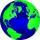How many different committees of 6 people can be formed from a class of 30 students?
2. Seating rules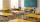In a class are 24 seats but in 7.B class are only 18 students. How many ways can student seat? (The class has 12 benches. A bench is for a pair of students.) Result (large number) logarithm and thus write down as powers of 10.
3. Colors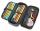Willie got birthday 6 colour pens in different colors. How many ways he can give them side by side in pencil?
4. Permutations without repetitionFrom how many elements we can create 720 permutations without repetition?
5. Cards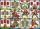How many ways can give away 32 playing cards to 5 player?
6. Value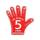Find the value of the expression: 6!·10^-3
7. CandiesIn the box are 12 candies that look the same. Three of them are filled with nougat, five by nuts, four by cream. At least how many candies must Ivan choose to satisfy itself that the selection of two with the same filling? ?
8. Theorem proveWe want to prove the sentence: If the natural number n is divisible by six, then n is divisible by three. From what assumption we started?
9. Complex number coordinatesWhich coordinates show the location of -2+3i
10. Virus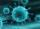We have a virus that lives one hour. Every half hour produce two child viruses. What will be the living population of the virus after 3.5 hours?
11. Gain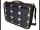Find the gain whenever the output power is 21x the input power.
12. Classroom 4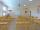In a class of 36 pupils, 2/3 are girls. How much it is in a class girls and boys?
13. MultiplesFind all multiples of 10 that are larger than 136 and smaller than 214.
14. CardsSuppose that are three cards in the hats. One is red on both sides, one of which is black on both sides, and a third one side red and the second black. We are pulled out of a hat randomly one card and we see that one side of it is red. What is the probabi
15. ShootersIn army regiment are six shooters. The first shooter target hit with a probability of 49%, next with 75%, 41%, 20%, 34%, 63%. Calculate the probability of target hit when shooting all at once.
16. VariableFind variable P: PP plus P x P plus P = 160
17. EquationEquation ? has one root x1 = 8. Determine the coefficient b and the second root x2.